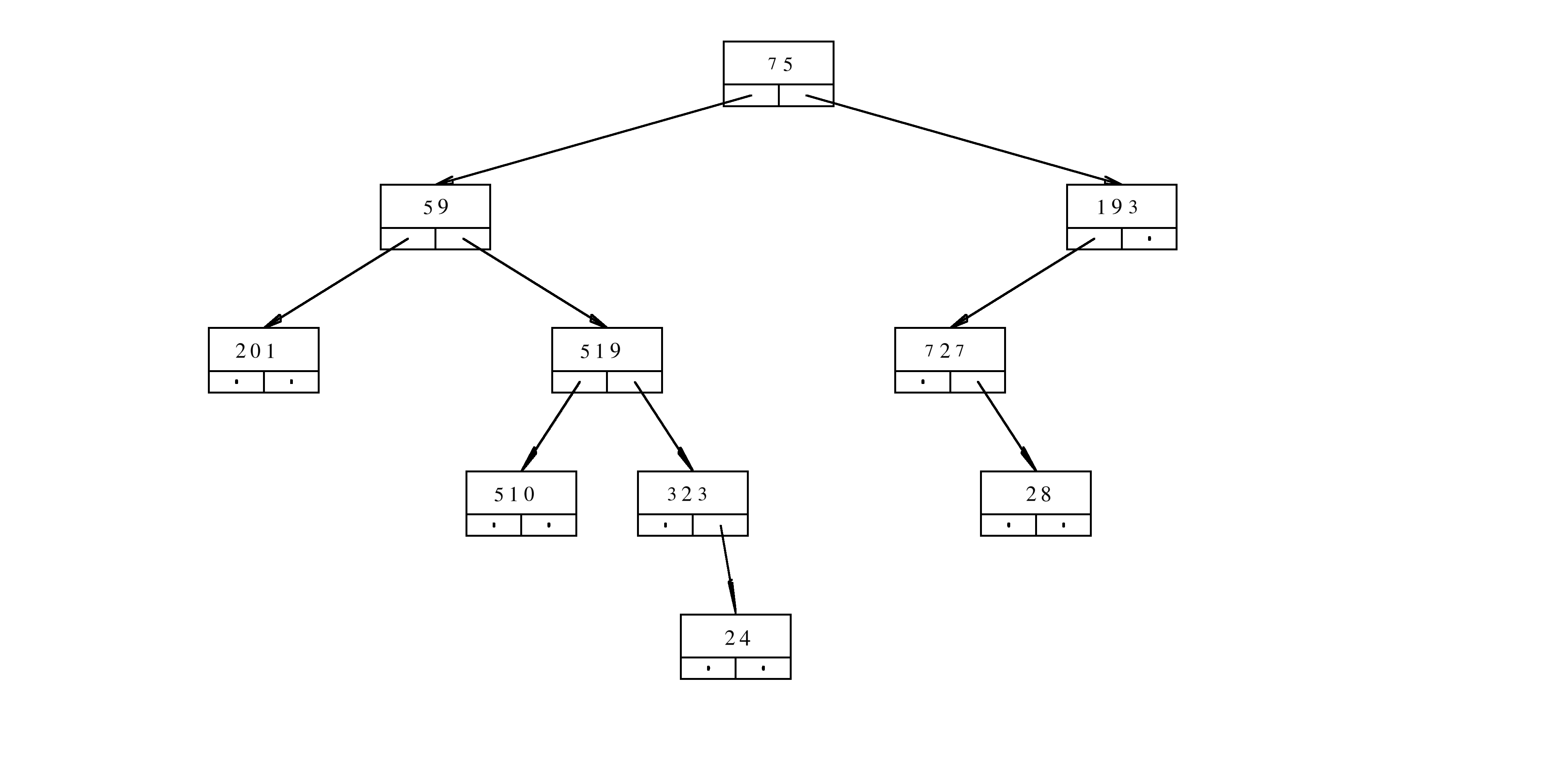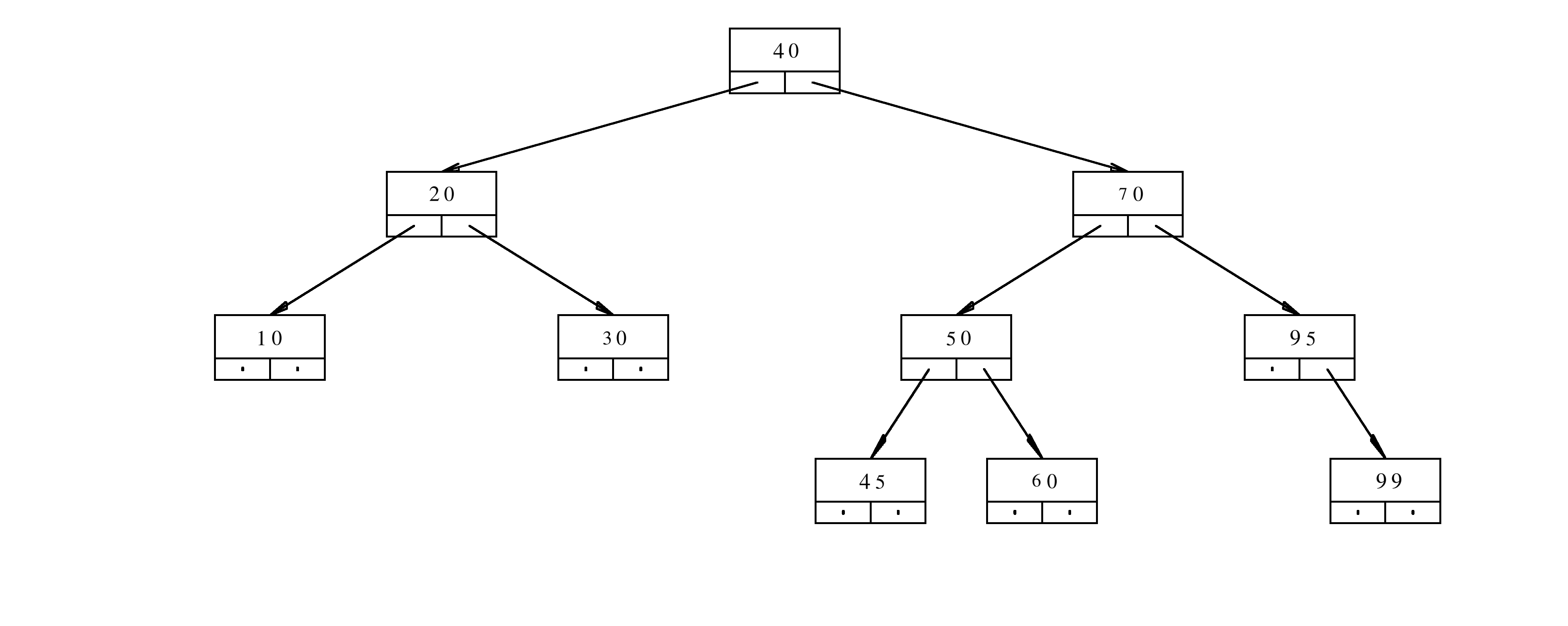MTH4300 Home

# Videos About Trees, Sets, and Maps

## Summary

The solution to the problem from the video 2:

## 1. Problems

Problem 1. The node of the binary tree is defined in the following way
class TN{
public:
long content;
TN* aLeft;
TN* aRight;
};
The implementation of the function f is given below
long f(TN* a, long h){
if(a==nullptr){
return 1;
}
long c=a->content;
return (c%10)+(h%2)*f(a->aLeft,h+1)+(c%2)*f(a->aRight,h+1);
}
Assume that aRoot is the pointer to the root of the treeWhat is the result of the evaluation f(aRoot,3)? Provide a rigorous justification for your answer.

Problem 2. What happens when the number $$55$$ is inserted in the following AVL tree? What is the resulting tree after all the rotations?